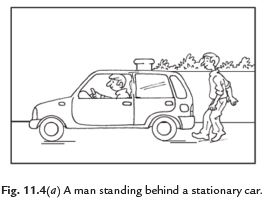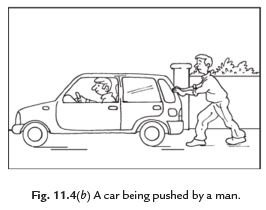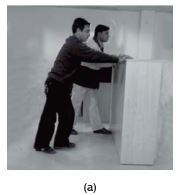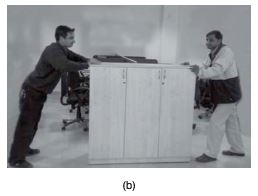Courses

# Short Answer Question - Force & Pressure Notes | EduRev

## Class 8 : Short Answer Question - Force & Pressure Notes | EduRev

The document Short Answer Question - Force & Pressure Notes | EduRev is a part of the Class 8 Course Science Class 8.
All you need of Class 8 at this link: Class 8

Q1: What is force? What is its unit?

Ans: A push or a pull on an object is called a force. It arises due to the interaction between two objects. Force has magnitude as well as direction. It may change in the state of motion of an object or it may bring about a change in the shape of an object. The unit of force is Newton.
Q2: What is change in state of motion? What brings change in state of motion?

Ans: A change in either the speed of an object, or its direction of motion, or both, is described as a change in its state of motion. Force may bring the change in the state of motion of an object.
Q3: What is the difference between contact forces and non-contact forces?

Ans: Forces which act only when there is physical contact between two interacting objects are known as Contact forces. Example: Muscular force. Whereas Forces which can act without physical contact between objects i.e. those that can act from a distance, are called non-contact forces or field forces. Example: Magnetic force.Magnetic Force Q4: Give two example each of the situation in which you apply force to change state of motion of an object and to change shape of an object?

Ans: A goal keeper applies force for saving a goal. By his action the goal keeper tries to apply a force on the moving ball. This leads to change in state of motion of ball.
Force is applied to the shape of a ball of dough when it is rolled to make a chapatti.
Q5: Why is it difficult to hold a school bag having a strap made of a thin and strong string?

Ans: It is difficult to hold a school bag having a strap made of a thin and strong string because it apply the large pressure on the shoulders due to very small contact surface area .The pressure is inversely proportional to the surface area on which the force acts. Therefore pressure decreases if surface area increases.
Q6: Why Porters wear turbans when they have to carry heavy loads on their heads?

Ans: Porters wear turbans when they have to carry heavy loads on their heads, to increase the area of contact. This reduces the pressure on the head.
Q7: Force applied on an object may change its speed. How?

Ans:  force applied on an object may change its speed. If the force applied on the object is in the direction of its motion, the speed of the object increases. If the force is applied in the direction opposite to the direction of motion, then it results in a decrease in the speed of the object
Q8: Why Lorries and trucks carrying heavy loads have 8 tyres instead of four?

Ans: Lorries and trucks carrying heavy loads have 8 tyres instead of four. Also the tyres are broader because this increases the area of contact with the ground, thus reducing the pressure exerted on the ground.
Q9: Why the sucker sticks to the surface over which it is pressed?

Ans: When we press the sucker, most of the air between its cup and the surface escapes out. The sucker sticks to the surface because pressure of atmosphere acts on it. To pull the sucker off the surface, the applied force should be large enough to overcome the atmospheric pressure.
Q10: What is pressure? How is pressure related to the surface area on which it acts?

Ans: Force per unit area is called pressure. Pressure is inversely proportional to the surface area on which it acts. Smaller the area, larger the pressure on the surface for the same force.
Q11: When we stop pedalling the bicycle, it slows down and gradually stops .Why?

Ans: When we stop pedalling the bicycle, it slows down and gradually stops because of force of friction between the surface of tyres of cycle and the ground that brings moving bicycle to rest. The direction of force of friction is always opposite to the direction of motion.
Q12: What is frictional force? How it arises?
Ans:
The force acting against the relative motion of surfaces in contact is called frictional force or friction. Friction is an example of contact force. Force of friction arises due to contact between surfaces.
Q13: What is magnetic force? What happens when we bring like poles of two magnets closer?

Ans: A magnet can exert force on another magnet without being in contact with it; this force is known as magnetic force. Like poles of two magnets repel each other. Unlike poles of two magnets attract each other.

Q14: What is gravitational force? Is gravity the property of earth alone?

Ans: Every object exerts a force on every other object. This force is known as gravitational force. Gravity is not a property of the earth alone. Every object in the universe whether large or small exerts gravitational force on every other object.

Q15: A rocket has been fired upwards to launch a satellite in its orbit. Name the two forces acting on the rocket just after leaving of the launching pad

Ans: The force acting on a rocket is:

• Frictional force due to air
• Force of gravity acting in downward direction.

Q16: What will be the effect of force while squeezing a piece of lemon between the fingers?

Ans: Agent exerting pressure: Fingers.

Object: Lemon.

Effect of force is observed: Lemon juice is coming out, change in shape of lemon.

Q17: What will be the effect of force while taking out toothpaste from toothpaste tube?

Ans: Agent exerting pressure: Fingers.
Object: Tooth paste tube
Effect of force is observed: Tooth paste is coming out, change in shape of tooth paste.
Q18: A blacksmith is hammering a hot piece of copper for making a tool, how does the force due to affect the piece of copper?

Ans: The force due to hammering changes the shape of the hot piece of copper to make tools.
Q19: What are the effects of the force while making high jump by an athlete?

Ans: Agent exerting pressure: An athlete.

Object: Body

Effect of force: Jumping
Q20: Differentiate between atmosphere and atmospheric pressure.

Ans: Atmosphere is the envelope of air surrounding our earth but the atmospheric pressure is the pressure exerted by this air.

Q21: Differentiate between friction and contact force.

Ans: Friction is the force responsible for changing the state of motion of objects but contact force is the forces which act only when there is physical contact between two interacting objects.
Q22: What is a force? Explain with the help of some examples.
Ans:
Force is a pull or push of the objects. The action like, pushing, pulling, picking, hitting, lifting, running and bending are the examples of force. Moving or stopping of a body, changing shape and direction of motion of objects are various actions which show force in play.

Q23: How do we feel force in our daily life?
Ans:
Various big or small actions make us feel the force. We hit or catch many objects in our daily life. We see that a moving ball stops on its own. The ball changes the direction of its motion when it is hit with a bat. We make lassi etc. by churning of curd. These are many actions which help us to feel that a force is exerted.

Q24: Explain that forces are due to an interaction between objects.
Ans:
Imagine, a man is standing behind a stationary car. The car does not move due to his presence.Now allow the man to push the car, it means he applies a force on the car. The car may begin to move in the direction of the applied force. Note that the man has to push the car to make it move. This example shows that at least two objects must interact with each other for a force to come into play.

Q25: What happens when (i) Two forces are exerted in same direction?
(ii) Two forces are exerted in opposite directions?
Ans:
(i) When two forces are exerted in same direction on an object, then the forces are added and action becomes easy.
(ii) When two forces act in the opposite directions on an object, the net force acting on it is the difference between the two forces.Fig. 11.5 Two friends pushing a heavy load (a) in the same direction, (b) in opposite directions.

Q26: What are the two factors on which effect of force depends?
Ans:
There are following two factors on which the effect of force depends:

(i) Magnitude: The strength of force is usually expressed by its magnitude.

(ii) Direction of force: We have also to specify the direction of force in which it acts.
If the direction or magnitude of force changes, its effect also changes.

Q27: What are the effects of force?
Ans:
A force changes or try to change the
(i) Speed of a moving body.
(ii) Direction of motion of a body.
(iii) Shape of a body.

Q28: What are states of motion?
Ans:
An  object can be in two positions, one is at rest or other in motion ; both are its states of motion. Any change in this position (rest or motion) can be called the change in the state of motion. The state of motion of an object is described by its speed and the direction of motion.

Q29: How can a force change the states of motion?
Ans:
There are two states of an object:

(i) Rest
(ii) Motion
When a force is applied on a body then it can move. In the same way a force can change the direction of a moving object as well as its speed. A force can stop a moving object.
If the force is applied in the direction of motion at an object, then its speed is increased. If the force acts in opposite direction, then the speed of the object is decreased.

Q30: What is the effect of force on the shape of an object?
Ans:
A force can change or try to change the shape of an object. When a force is applied on an object then change in shape takes place. It may be smaller or greater. At last we can say that the application of force on an object may change its shape.

Q31: Explain contact and non-contact forces.
Ans:
Contact forces: The forces which come into play only when two objects come in contact with each other are called contact forces. Muscular force and force of friction are the examples of contact forces.
Non-contact forces: The forces which come into play without any contact of objects with one another are called non-contact forces. Electrostatic and magnetic forces are the examples of non-contact forces.

Q32: What is muscular force? Why is it called contact force?
Ans:
The force resulting due to the action of muscles is known as the muscular force. Muscular force is called contact force because it comes in action only when two bodies come in contact with each other.

Q33: What do you understand by the force of friction?
Ans.
The force which always acts on all the moving objects and whose direction is always opposite to the direction of motion is called force of friction. Since the force of friction arises due to contact between surfaces, it is also called contact force.

Q 34: What is electrostatic force? Why is it called non-contact force?
Ans:
The force exerted by a charged body on another charged or uncharged body is called electrostatic force. This force comes into play even when the bodies are not in contact, so it is called non-contact force.

Q35: Explain force of gravity.
Ans:
Objects or the things that fall towards the earth because the earth pulls them. This force is called force of gravity or the force due to gravitation. This is also called just gravity. It is an attractive force. This force acts on all objects.

Q36: What is pressure? What happens to the pressure when area on which it is applied increases?
Ans:
The force acting on a unit area of a surface is called pressure.
Pressure = Force/Area on which it acts.
The pressure is inversely proportional to the area on which force is applied. As the area on which force is applied is increased, the pressure decreases. We can say that pressure increases with decrease in area.

Q37: We observe that the wheels of buses and trucks are heavier than the wheels of cars or scooters. Why?
Ans:
The buses and trucks are heavy and require to exert less pressure. We know that pressure decreases as area of contact increases, so the tyres are broadened so that they exert less pressure on earth and move easily. If they exert more pressure, they will sink in earth. The scooters and cars have less wide wheels because they need more pressure for gripping.
Q38: What is atmospheric pressure?
Ans:
The envelop of air around the earth is known as atmosphere. The atmospheric air extends up to many kilometres above the surface of the earth. The pressure exerted by the air is called atmospheric pressure.

Q39: If the area of your head is 15 cm × 15 cm, how much air (in weight) would you carry on your head?
Ans:
The weight of air column of the height of the atmosphere and area 15 cm × 15 cm is equal to  the  weight  of  an  object  of  mass  225  kg  (2250  N).  We  have  air  inside  our body,  so  we  are  not crushed under this weight and pressure of air is equal at both the sides.

Offer running on EduRev: Apply code STAYHOME200 to get INR 200 off on our premium plan EduRev Infinity!

## Science Class 8

143 videos|244 docs|81 tests

,

,

,

,

,

,

,

,

,

,

,

,

,

,

,

,

,

,

,

,

,

;